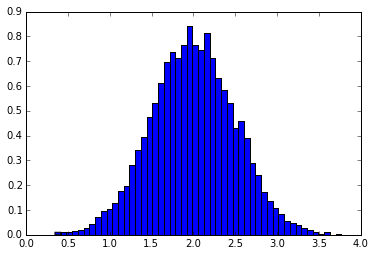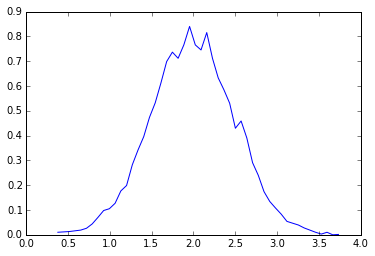# Lesson 8 - Tricks and Tips¶

Here we give a list of short and useful tips

In :
import numpy as np


## "Automatic" Reshaping¶

To change the dimensions of an array, you can omit one of the sizes which will then be deduced automatically:

In :
a = np.arange(30)

In :
a

Out:
array([ 0,  1,  2,  3,  4,  5,  6,  7,  8,  9, 10, 11, 12, 13, 14, 15, 16,
17, 18, 19, 20, 21, 22, 23, 24, 25, 26, 27, 28, 29])
In :
a.shape = 2,-1,3 # -1 means "whatever is needed"

In :
a

Out:
array([[[ 0,  1,  2],
[ 3,  4,  5],
[ 6,  7,  8],
[ 9, 10, 11],
[12, 13, 14]],

[[15, 16, 17],
[18, 19, 20],
[21, 22, 23],
[24, 25, 26],
[27, 28, 29]]])
In :
a.shape

Out:
(2L, 5L, 3L)

## Vector Stacking¶

How do we construct a 2D array from a list of equally-sized row vectors? In MATLAB this is quite easy: if x and y are two vectors of the same length you only need do m=[x;y]. In NumPy this works via the functions column_stack, dstack, hstack and vstack, depending on the dimension in which the stacking is to be done. For example:

In :
x = np.arange(0,10,2)      # x = ([0,2,4,6,8])
y = np.arange(5)           # y = ([0,1,2,3,4])
m = np.vstack([x,y])       # m = ([[0,2,4,6,8],
#       [0,1,2,3,4]])
xy = np.hstack([x,y])      # xy = ([0,2,4,6,8,0,1,2,3,4])

In :
x

Out:
array([0, 2, 4, 6, 8])
In :
y

Out:
array([0, 1, 2, 3, 4])
In :
m

Out:
array([[0, 2, 4, 6, 8],
[0, 1, 2, 3, 4]])
In :
xy

Out:
array([0, 2, 4, 6, 8, 0, 1, 2, 3, 4])

## Histograms¶

The NumPy histogram function applied to an array returns a pair of vectors: the histogram of the array and the vector of bins. Beware: matplotlib also has a function to build histograms (called hist, as in Matlab) that differs from the one in NumPy. The main difference is that pylab.hist plots the histogram automatically, while numpy.histogram only generates the data.

Note: see this post for information on displaying plots inline within the IPython Notebook.

In :
# This line configures matplotlib to show figures embedded in the notebook,
# instead of opening a new window for each figure. More about that later.
%pylab inline

Populating the interactive namespace from numpy and matplotlib

In :
import pylab

In :
# Build a vector of 10000 normal deviates with variance 0.5^2 and mean 2
mu, sigma = 2, 0.5
v = np.random.normal(mu,sigma,10000)

In :
# Plot a normalized histogram with 50 bins
pylab.hist(v, bins=50, normed=1) # matplotlib version (plot)

Out:
(array([ 0.01022988,  0.01169129,  0.0131527 ,  0.01607552,  0.01899834,
0.0263054 ,  0.04384233,  0.07014773,  0.09791454,  0.10522159,
0.12714276,  0.17683073,  0.1987519 ,  0.28205232,  0.34197017,
0.39604238,  0.47349716,  0.5319536 ,  0.61233121,  0.69855445,
0.73655114,  0.71170715,  0.76577936,  0.84031132,  0.76577936,
0.74531961,  0.81546733,  0.71170715,  0.63279096,  0.5845644 ,
0.53049219,  0.42965483,  0.45888305,  0.39019674,  0.29082079,
0.2396714 ,  0.17390791,  0.13444981,  0.10814441,  0.08330043,
0.05407221,  0.04676515,  0.0394581 ,  0.02776681,  0.01899834,
0.01022988,  0.00292282,  0.01022988,  0.        ,  0.00146141]),
array([ 0.34679319,  0.41522021,  0.48364723,  0.55207425,  0.62050127,
0.6889283 ,  0.75735532,  0.82578234,  0.89420936,  0.96263638,
1.0310634 ,  1.09949042,  1.16791744,  1.23634446,  1.30477148,
1.3731985 ,  1.44162552,  1.51005254,  1.57847956,  1.64690658,
1.7153336 ,  1.78376062,  1.85218764,  1.92061466,  1.98904169,
2.05746871,  2.12589573,  2.19432275,  2.26274977,  2.33117679,
2.39960381,  2.46803083,  2.53645785,  2.60488487,  2.67331189,
2.74173891,  2.81016593,  2.87859295,  2.94701997,  3.01544699,
3.08387401,  3.15230103,  3.22072805,  3.28915508,  3.3575821 ,
3.42600912,  3.49443614,  3.56286316,  3.63129018,  3.6997172 ,
3.76814422]),
<a list of 50 Patch objects>)In :
pylab.show()

In :
# Compute the histogram with numpy and then plot it
(n, bins) = numpy.histogram(v, bins=50, normed=True) # NumPy version (no plot)

In :
pylab.plot(.5*(bins[1:]+bins[:-1]), n)

Out:
[<matplotlib.lines.Line2D at 0x9f0ddd8>]In :
pylab.show()


# Conclusion¶

We have now completed lesson 8 Tricks and Tips.